Home > CCA2 > Chapter 11 > Lesson 11.2.1 > Problem11-49

11-49.
1. Logarithms are used to measure the “loudness” of sound. Decibels (dB) are logarithmic units used to describe a ratio of two levels of intensity or pressure. The difference between two levels of sound pressure ( P1 and P2 ) is defined as 10 log(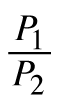)dB. Usually, when decibels are used to describe just one sound, it is assumed that that sound is being compared to a reference level of 20 micropascals. Homework Help ✎

1. How many decibels correspond to doubling the pressure of a sound?

2. What is the sound pressure of a noise described as 60 dB?

3. What does 0 decibels mean?

4. How many times more pressure is in a sound of 40 dB than of 20 dB?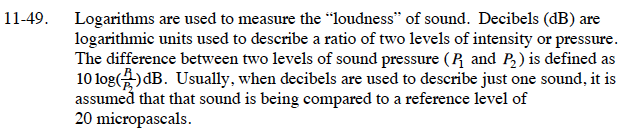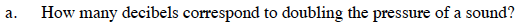10 log(2) ≈ 3.0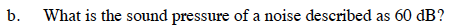$10\cdot \text{log}\left(\frac{P}{20}\right)=60$

$\text{log}\left(\frac{P}{20}\right)=6$

$10^6=\frac{P}{20}$

P = 20 · 106 = 2 · 107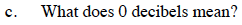The sound ratio is 1.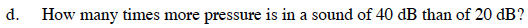100 times more pressure.